# Graph Match

## Match the graphs with their equations or descriptions. A self-marking, drag-and-drop mathematical exercise.

##### GradientLevel 1Level 2Level 3Level 4Exam-StyleDescriptionHelpMore Graph Activities

This is Level 1 (Linear graphs and equations). Match the graphs with the corresponding equations.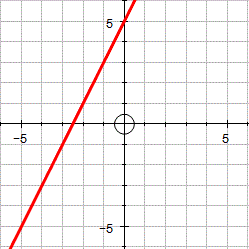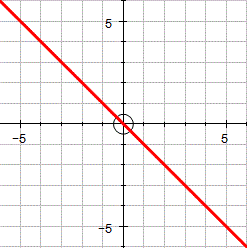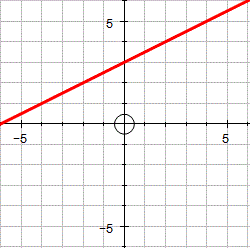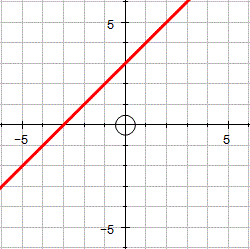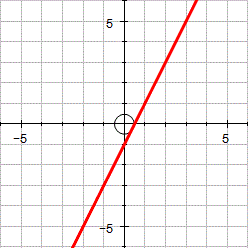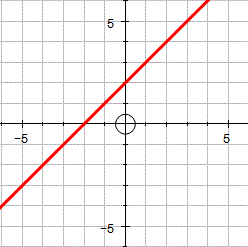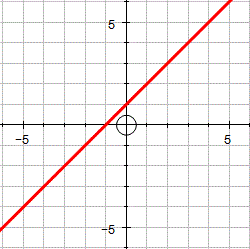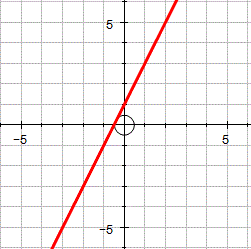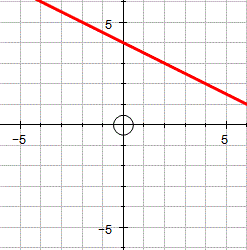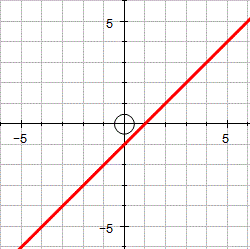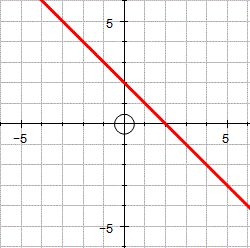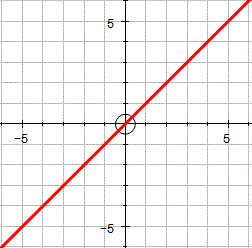$$y = 2x + 5$$

$$y = - x$$

$$y = \frac12 x + 3$$

$$y = x + 3$$

$$y = 2x − 1$$

$$y = x + 2$$

$$y = x + 1$$

$$y = 2x + 1$$

$$y = 4 - \frac12 x$$

$$y = x - 1$$

$$y = 2-x$$

$$y = x$$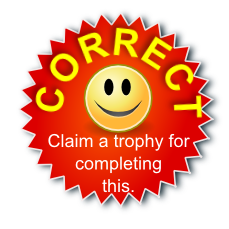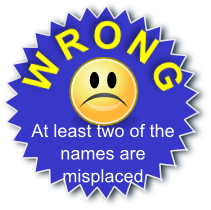The diagrams were created in Autograph.

This is Graph Match level 1. You can also try:
Gradient Level 2 Level 3 Level 4 Exam-style Questions

## Description of Levels

CloseGradient - A pre-requisite for doing the graph exercises is being able to calculate the gradient of a line.

Level 1 - Linear graphs and equations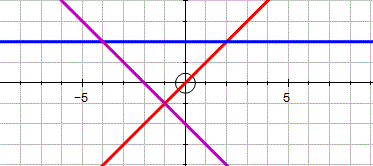Level 2 - Linear and quadratic graphs and equations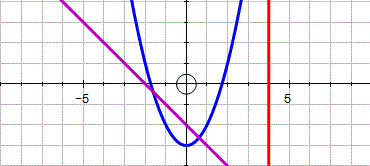Level 3 - Mixed polynomials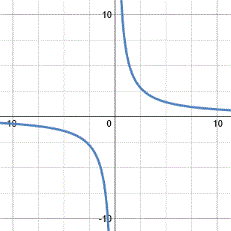Level 4 - Quadratics in the form $$ax^2 + bx + c$$ given information about the coefficients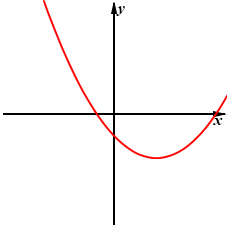Exam Style questions are in the style of GCSE or IB/A-level exam paper questions and worked solutions are available for Transum subscribers.

More on this topic including lesson Starters, visual aids and investigations.

Answers to this exercise are available lower down this page when you are logged in to your Transum account. If you don’t yet have a Transum subscription one can be very quickly set up if you are a teacher, tutor or parent.

For straight line graphs arrange the equation in the form $$y = mx + c$$ where $$m$$ represents the gradient of the line and $$c$$ the y-intercept.

Maybe this video will remind you of some of the techniques for recognising graphs.

This video is from the ukmathsteacher YouTube channel.

Answers to this exercise are available lower down this page when you are logged in to your Transum account. If you don’t yet have a Transum subscription one can be very quickly set up if you are a teacher, tutor or parent.

Close## Transum.org

This web site contains over a thousand free mathematical activities for teachers and pupils. Click here to go to the main page which links to all of the resources available.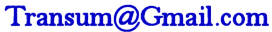## More Activities:

Mathematicians are not the people who find Maths easy; they are the people who enjoy how mystifying, puzzling and hard it is. Are you a mathematician?

Comment recorded on the 24 May 'Starter of the Day' page by Ruth Seward, Hagley Park Sports College:

"Find the starters wonderful; students enjoy them and often want to use the idea generated by the starter in other parts of the lesson. Keep up the good work"

Comment recorded on the 9 May 'Starter of the Day' page by Liz, Kuwait:

"I would like to thank you for the excellent resources which I used every day. My students would often turn up early to tackle the starter of the day as there were stamps for the first 5 finishers. We also had a lot of fun with the fun maths. All in all your resources provoked discussion and the students had a lot of fun."

#### Nine Digits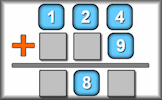Arrange the given digits one to nine to make three numbers such that two of them add up to the third. This is a great puzzle for practicing standard pen and paper methods of three digit number addition and subtraction.

There are answers to this exercise but they are available in this space to teachers, tutors and parents who have logged in to their Transum subscription on this computer.

A Transum subscription unlocks the answers to the online exercises, quizzes and puzzles. It also provides the teacher with access to quality external links on each of the Transum Topic pages and the facility to add to the collection themselves.

Subscribers can manage class lists, lesson plans and assessment data in the Class Admin application and have access to reports of the Transum Trophies earned by class members.

Subscribe

## Go Maths

Learning and understanding Mathematics, at every level, requires learner engagement. Mathematics is not a spectator sport. Sometimes traditional teaching fails to actively involve students. One way to address the problem is through the use of interactive activities and this web site provides many of those. The Go Maths main page links to more activities designed for students in upper Secondary/High school.

## Teachers

If you found this activity useful don't forget to record it in your scheme of work or learning management system. The short URL, ready to be copied and pasted, is as follows:

Do you have any comments? It is always useful to receive feedback and helps make this free resource even more useful for those learning Mathematics anywhere in the world. Click here to enter your comments.For All: